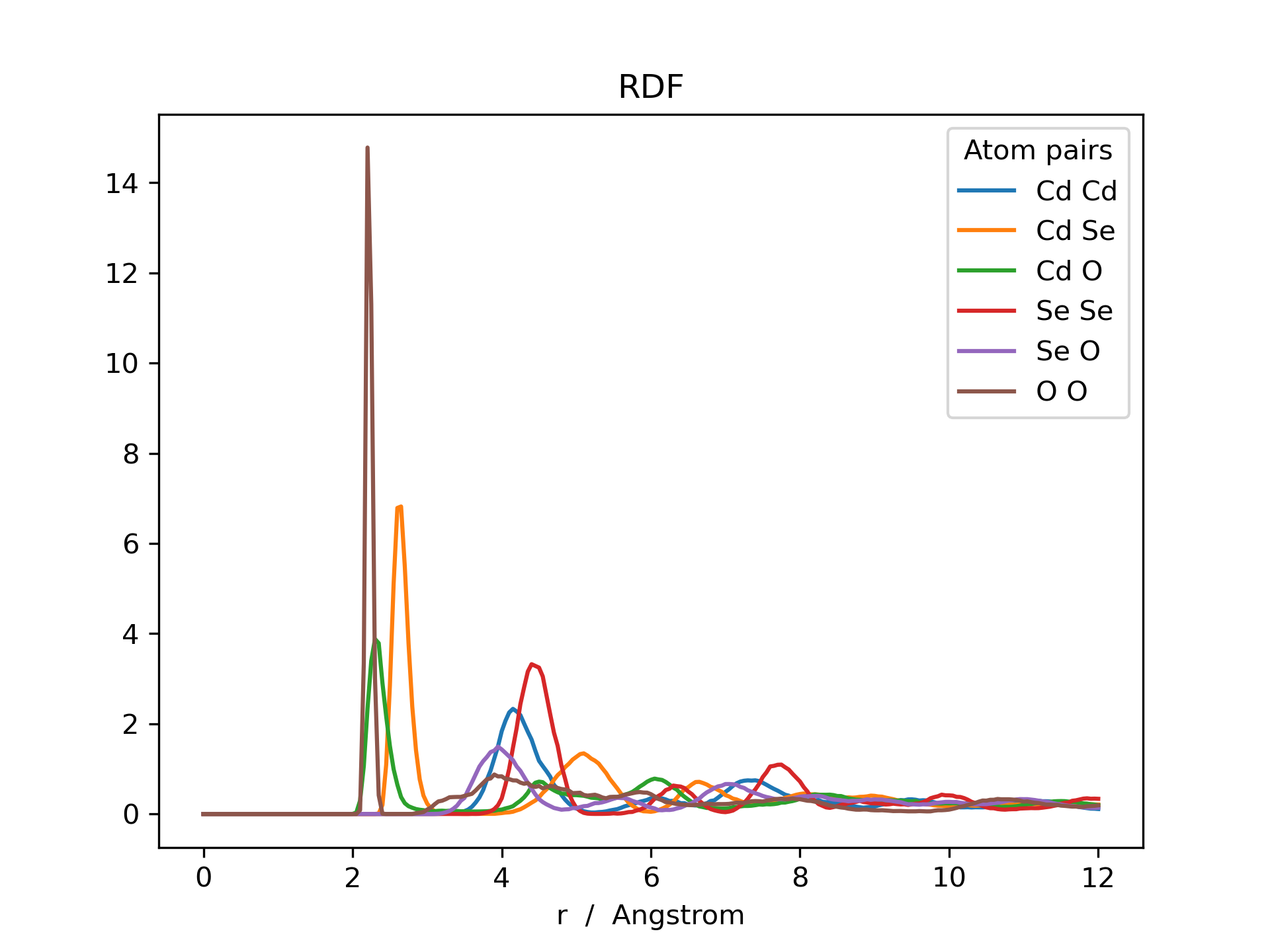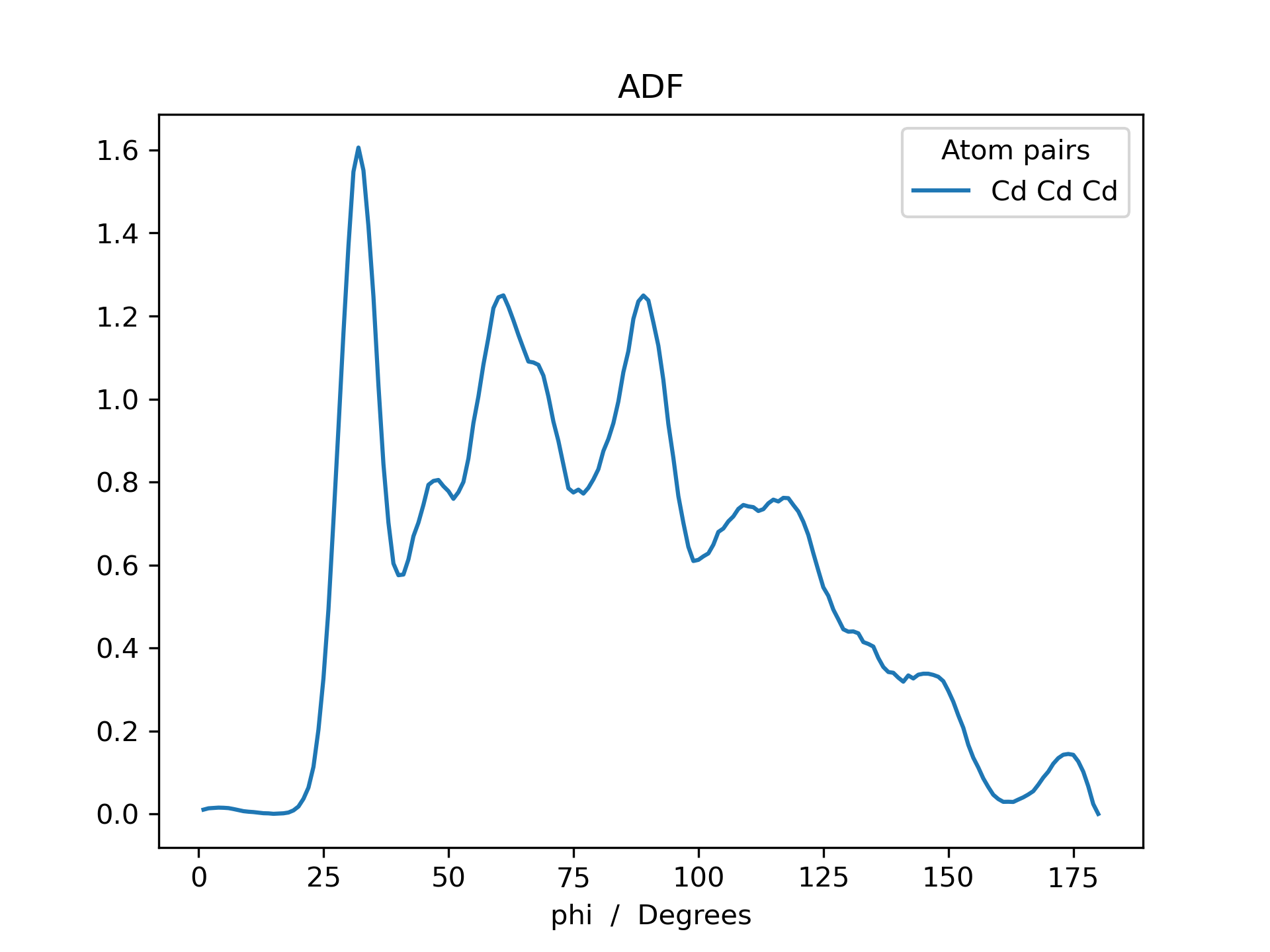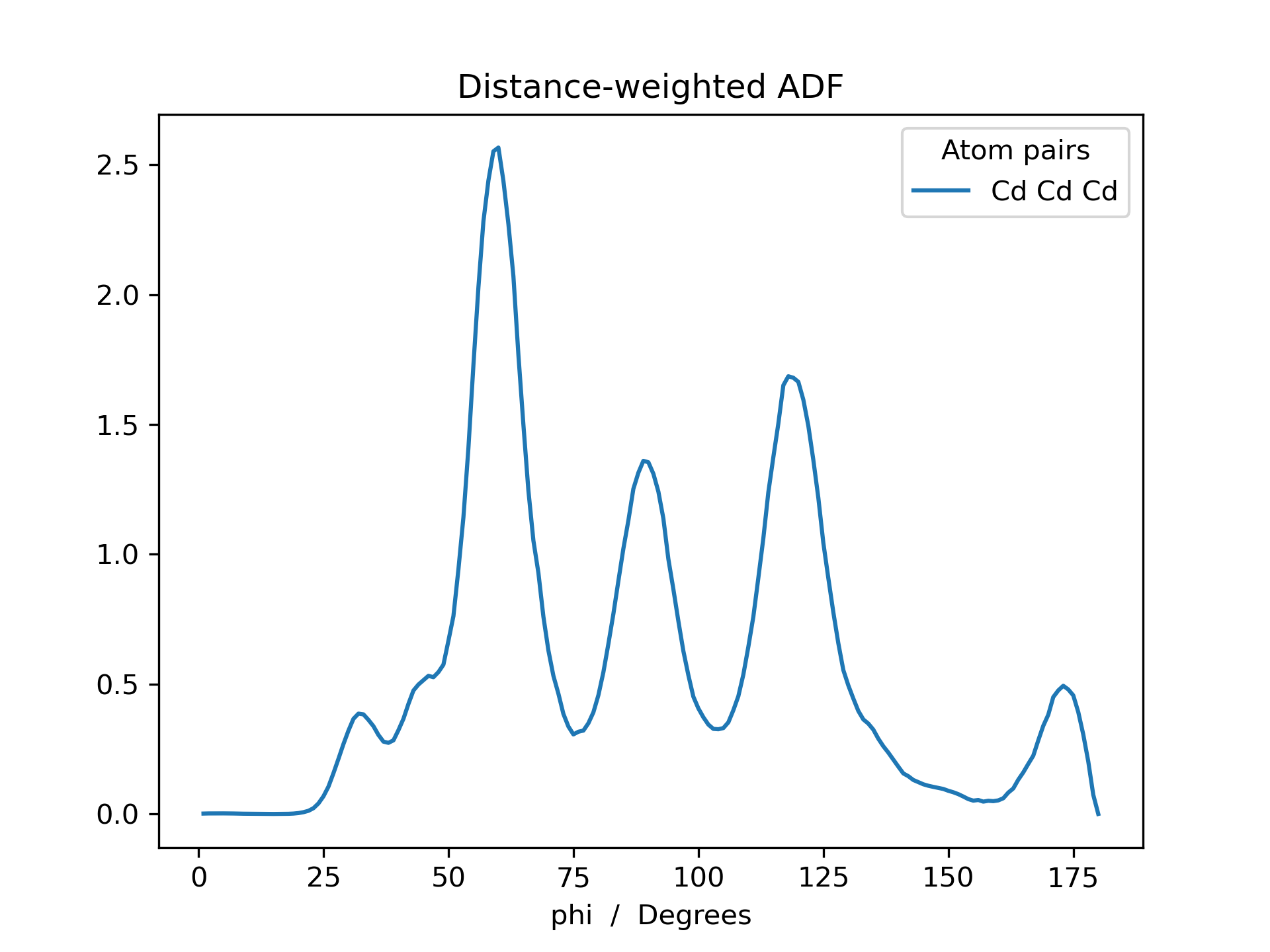# Radial & Angular Distribution Function¶

Radial and angular distribution function (RDF & ADF) generators have been implemented in the FOX.MultiMolecule class. The radial distribution function, or pair correlation function, describes how the particale density in a system varies as a function of distance from a reference particle. The herein implemented function is designed for constructing RDFs between all possible (user-defined) atom-pairs.

$g(r) = \frac{V}{N_a*N_b} \sum_{i=1}^{N_a} \sum_{j=1}^{N_b} \left< *placeholder* \right>$

Given a trajectory, mol, stored as a FOX.MultiMolecule instance, the RDF can be calculated with the following command: rdf = mol.init_rdf(atom_subset=None, low_mem=False). The resulting rdf is a Pandas dataframe, an object which is effectively a hybrid between a dictionary and a NumPy array.

A slower, but more memory efficient, method of RDF construction can be enabled with low_mem=True, causing the script to only store the distance matrix of a single molecule in memory at once. If low_mem=False, all distance matrices are stored in memory simultaneously, speeding up the calculation but also introducing an additional linear scaling of memory with respect to the number of molecules. Note: Due to larger size of angle matrices it is recommended to use low_mem=False when generating ADFs.

Below is an example RDF and ADF of a CdSe quantum dot pacified with formate ligands. The RDF is printed for all possible combinations of cadmium, selenium and oxygen (Cd_Cd, Cd_Se, Cd_O, Se_Se, Se_O and O_O).

>>> from FOX import MultiMolecule, example_xyz

>>> mol = MultiMolecule.from_xyz(example_xyz)

# Default weight: np.exp(-r)
>>> rdf = mol.init_rdf(atom_subset=('Cd', 'Se', 'O'))
>>> adf = mol.init_adf(r_max=8, weight=None, atom_subset=('Cd',))
>>> adf_weighted = mol.init_adf(r_max=8, atom_subset=('Cd',))

>>> rdf.plot(title='RDF')
>>> adf.plot(title='ADF')
>>> adf_weighted.plot(title='Distance-weighted ADF')One can take into account a systems periodicity by settings the molecules’ lattice vectors and specifying the axes along which the system is periodic.

The lattice vectors can be provided in one of two formats:

• A $$(3, 3)$$ matrix.
• A $$(N_{mol}, 3, 3)$$-shaped tensor if they vary across the trajectory.
>>> from FOX import MultiMolecule
>>> import numpy as np

>>> lattice = np.array(...)
>>> mol = MultiMolecule.from_xyz(...)
>>> mol.lattice = lattice

# Periodic along the x, y and/or z axes
>>> rdf = mol.init_rdf(atom_subset=('Cd', 'Se', 'O'), periodic="xy")
>>> adf = mol.init_adf(r_max=8, atom_subset=('Cd',), periodic="xyz")


## API¶

MultiMolecule.init_rdf(mol_subset=None, atom_subset=None, *, dr=0.05, r_max=12.0, periodic=None)[source]

Initialize the calculation of radial distribution functions (RDFs).

RDFs are calculated for all possible atom-pairs in atom_subset and returned as a dataframe.

Parameters: mol_subset (slice, optional) – Perform the calculation on a subset of molecules in this instance, as determined by their moleculair index. Include all $$m$$ molecules in this instance if None. atom_subset (Sequence[str], optional) – Perform the calculation on a subset of atoms in this instance, as determined by their atomic index or atomic symbol. Include all $$n$$ atoms per molecule in this instance if None. dr (float) – The integration step-size in Ångström, i.e. the distance between concentric spheres. r_max (float) – The maximum to be evaluated interatomic distance in Ångström. periodic (str, optional) – If specified, correct for the systems periodicity if self.lattice is not None. Accepts "x", "y" and/or "z". A dataframe of radial distribution functions, averaged over all conformations in xyz_array. Keys are of the form: at_symbol1 + ‘ ‘ + at_symbol2 (e.g. "Cd Cd"). Radii are used as index. pd.DataFrame
MultiMolecule.init_adf(mol_subset=None, atom_subset=None, r_max=8.0, weight=<function neg_exp>, periodic=None)[source]

Initialize the calculation of distance-weighted angular distribution functions (ADFs).

ADFs are calculated for all possible atom-pairs in atom_subset and returned as a dataframe.

Parameters: mol_subset (slice, optional) – Perform the calculation on a subset of molecules in this instance, as determined by their moleculair index. Include all $$m$$ molecules in this instance if None. atom_subset (Sequence[str], optional) – Perform the calculation on a subset of atoms in this instance, as determined by their atomic index or atomic symbol. Include all $$n$$ atoms per molecule in this instance if None. r_max (float) – The maximum inter-atomic distance (in Angstrom) for which angles are constructed. The distance cuttoff can be disabled by settings this value to np.inf, "np.inf" or "inf". weight (Callable[[np.ndarray], np.ndarray], optional) – A callable for creating a weighting factor from inter-atomic distances. The callable should take an array as input and return an array. Given an angle $$\phi_{ijk}$$, to the distance $$r_{ijk}$$ is defined as $$max[r_{ij}, r_{jk}]$$. Set to None to disable distance weighting. periodic (str, optional) – If specified, correct for the systems periodicity if self.lattice is not None. Accepts "x", "y" and/or "z". A dataframe of angular distribution functions, averaged over all conformations in this instance. pd.DataFrame

Note

Disabling the distance cuttoff is strongly recommended (i.e. it is faster) for large values of r_max. As a rough guideline, r_max="inf" is roughly as fast as r_max=15.0 (though this is, of course, system dependant).

Note

The ADF construction will be conducted in parralel if the DASK package is installed. DASK can be installed, via anaconda, with the following command: conda install -n FOX -y -c conda-forge dask.

FOX.recipes.time_resolved_rdf(mol, start=0, stop=None, step=500, **kwargs)[source]

Calculate the time-resolved radial distribution function (RDF).

Examples

>>> from FOX import MultiMolecule, example_xyz
>>> from FOX.recipes import time_resolved_rdf

# Calculate each RDF over the course of 500 frames
>>> time_step = 500
>>> mol = MultiMolecule.from_xyz(example_xyz)

>>> rdf_list = time_resolved_rdf(
...     mol, step=time_step, atom_subset=['Cd', 'Se']
... )

Parameters: mol (MultiMolecule) – The trajectory in question. start (int) – The initial frame. stop (int, optional) – The final frame. Set to None to iterate over all frames. step (int) – The number of frames per individual RDF. Note that lower step values will result in increased numerical noise. **kwargs (Any) – Further keyword arguments for init_rdf(). A list of dataframes, each containing an RDF calculated over the course of step frames. List[pandas.DataFrame]

See also

init_rdf()
Calculate the radial distribution function.
FOX.recipes.time_resolved_rdf(mol, start=0, stop=None, step=500, **kwargs)[source]

Calculate the time-resolved radial distribution function (RDF).

Examples

>>> from FOX import MultiMolecule, example_xyz
>>> from FOX.recipes import time_resolved_rdf

# Calculate each RDF over the course of 500 frames
>>> time_step = 500
>>> mol = MultiMolecule.from_xyz(example_xyz)

>>> rdf_list = time_resolved_rdf(
...     mol, step=time_step, atom_subset=['Cd', 'Se']
... )

Parameters: mol (MultiMolecule) – The trajectory in question. start (int) – The initial frame. stop (int, optional) – The final frame. Set to None to iterate over all frames. step (int) – The number of frames per individual RDF. Note that lower step values will result in increased numerical noise. **kwargs (Any) – Further keyword arguments for init_rdf(). A list of dataframes, each containing an RDF calculated over the course of step frames. List[pandas.DataFrame]

See also

init_rdf()
Calculate the radial distribution function.